Courses

# Quantitative Aptitude - Test 5

## 25 Questions MCQ Test SSC CGL Tier 1 Mock Test Series | Quantitative Aptitude - Test 5

Description
This mock test of Quantitative Aptitude - Test 5 for SSC helps you for every SSC entrance exam. This contains 25 Multiple Choice Questions for SSC Quantitative Aptitude - Test 5 (mcq) to study with solutions a complete question bank. The solved questions answers in this Quantitative Aptitude - Test 5 quiz give you a good mix of easy questions and tough questions. SSC students definitely take this Quantitative Aptitude - Test 5 exercise for a better result in the exam. You can find other Quantitative Aptitude - Test 5 extra questions, long questions & short questions for SSC on EduRev as well by searching above.
QUESTION: 1

### A is thrice as fast as B and is therefore able to finish a work in 40 days less than that of B. Find the time in which they can do it working together.

Solution:

Given,
Ratio of time taken by A and B = 1 : 3
∴ B takes 3 days to finish a unit work while A takes 1 day to finish the same work
The difference of time is 2 days
Given,
Difference of time is 40 days
∴ Time taken by B to complete the work = [(3/2) × 40] = 60 days
∴ A takes 20 days to finish a work
⇒ Work in 1 day by A = 1/20
⇒ Work in 1 day by B = 1/60
⇒ Work in 1 day by (A+B) = [(1/20) + (1/60)] = 4/60
∴ A and B will take days to complete the work = 15 days

QUESTION: 2

### Area of the circle inscribed in a square of diagonal 4 cm is

Solution:

Let, the side of square be a
Given,
Diagonal of square (d) = 4 cm
⟹ Side of square = Diameter of inscribed circle
Using Pythagoras theorem:
⟹ d2 = a2 + a2
⟹ (4)2 = 2a2
⟹ a = 2√2cm
∴ Radius of circle = √2cm
⟹ Area of circle = π × r2
∴ Area of circle = π × (√2)2 = 2π

QUESTION: 3

### The original price of a TV set is Rs. 4,000.If the price is discounted by 10% and then raised by 5% for service contract, the price charged by the shopkeeper is

Solution:

Original price of a TV set = Rs. 4,000
∴ after 10% discount price will be = [4000 - (10/100) × 4000] = Rs. 3600
Given,
Increase in service contract = 5%
∴ The raised 5% service contract on new price will be = (5/100) × 3600 = Rs. 180
∴ Final selling price = 3600 + 180 = Rs. 3780

QUESTION: 4

A certain sum of money was divided between A, B and C in the ratio 1 : 3 : 5.If A received Rs. 500 the sum divided was

Solution:

Let, the distributed amount among A, B and C are x, 3x and 5x respectively
Given,
‘A’ received amount = Rs. 500
∴ x = 500
⟹ ‘B’ received amount = Rs. (500 × 3) = Rs. 1500
⟹ ‘C’ received amount = Rs. (500 × 5) = Rs. 2500
⟹ Sum of money = Rs. (500 + 1500 + 2500) = Rs. 4500

QUESTION: 5

By selling a bag at Rs. 300, profit of 10% is made. The selling price of the bag, when it is sold at 15% profit would be

Solution:

Given,
S.P1 = Rs.300, Profit1 = 10% and Profit2 = 15%
We know that,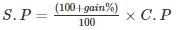Where, S.P = Selling Price, C.P = Cost Price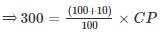⟹ C.P = Rs. 272.72
⟹ ProfitProfit%/100 × C.P
⟹ Profit2 = 15/100 × 272.72
⟹ Profit2 ≈ Rs. 41
⟹ SP2 = C.P + Profit2
⟹ SP2 = 272.72 + 41
∴ Selling price of the bag will be = Rs. 313.72

QUESTION: 6

The weights of two iron balls are 5 kg and 7 kg. What is the percentage weight of the 1st ball with respect to 2nd ball.

Solution:

We know that,
Percentage weight of 1st ball with respect to 2nd ball will be = 5/7 × 100 = 71 3/7 %

QUESTION: 7

A Bus travels at the speed of 40 km/hr, then the distance covered by it in 5 second is

Solution:

Given,
Speed of bus = 40 km/hr = (100/9) m/s
Time = 5 s
We know that,
Distance = Speed × time
∴ Distance = (100/9) × 5 = 55.55 m

QUESTION: 8

Find the value of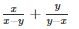Solution: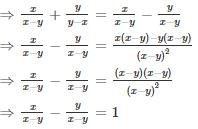QUESTION: 9

The value of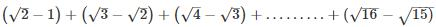Solution:

On solving,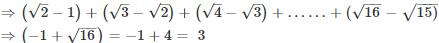QUESTION: 10

∆XYZ and ∆PQR are two similar triangles and the perimeter of ∆XYZ and ∆PQR are 160 cm and 40 cm respectively. If length of PQ = 20 cm, then length of XY is

Solution:

Let, length of XY be x
Given,
Perimeter (P1) of ∆XYZ = 160 cm
Perimeter (P2) of ∆PQR = 40 cm
∵ ∆XYZ and ∆PQR are similar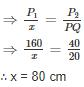QUESTION: 11

If the length of a chord of a circle is equal to that of the radius of the circle, then the angle subtended, in radians, at the center of the circle by chord is

Solution:

Given,
Chord length = radius of circle = r
Draw a perpendicular line of length x from center O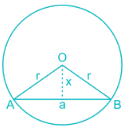Using Pythagoras theorm,
OA2 = Aa2 + Oa2
⇒ r2 = (r/2)2 + x2
⇒ x2 = 3r2/4
⇒ x = √3/2 r
Take any one triangle so formed
⟹ cos θ = √3/2
⟹ θ = π/6
∴ The angle subtended by the chord at the center = 2 × π/6 = π/3

QUESTION: 12

The value of (sin60° - cosec2 45°) + (cot2 30° + tan45°)

Solution:

We know that,
sin 60° = √3/2
cosec 45° = √2
cot 30° = √3
tan 45° = 1
Putting these values in the given equation,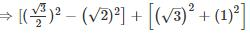⟹ [(3/4) – 2] + [3 + 1] = 11/4

QUESTION: 13

The average salary of male employees in a firm was Rs. 6000 and that of females was Rs. 5600. The mean salary of all the employees was Rs. 5800. What is the % of female employees?

Solution:

Let, the number of males be ‘m’ and number of females be ‘n’
Salaries of male employees be (x1, x2, x3,…….xm)
Salaries of females employees be (y1, y2, y3,………yn)
Given,
Average salary of males = Rs.6000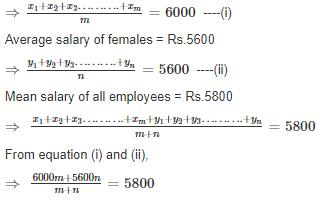⟹ m/n = 1
⟹ m = n  ----(iii)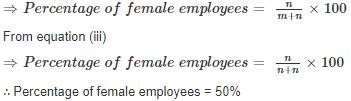QUESTION: 14

If x +1 = √3, then the value of x2+2√3

Solution:

Given,
x = √3-1
Put the value of x in given equation
⇒ x2 + 2√3 = (√3 - 1)2 + 2√3
⇒ 3 + 1 - 2√3 + 2√3 = 4

QUESTION: 15

The cube of 55 is

Solution:

We can write,
(55)= (50 + 5)3
We know that,
(a + b)3 = (a)3 + (b)3 + 3 × (a × b) (a + b)
⟹ (50 + 5)= (50)3 + (5)3 + 3 × (50 × 5) (50 + 5)
⟹ (50 + 5)3 = (125000) + (125) + 3 × 250 × 55
∴ (50 + 5)3 = 166375

QUESTION: 16

In ∆PQR, ∠Q is right angle, S is the mid-point of the side PR. If PQ = 4 cm, QR = 6 cm, then the length of PS is

Solution:

Using Pythagoras's theorem,
⇒ PR2 = PQ2 + QR2
⇒ PR2 = 42 + 62
⇒ PR = √52 cm = 2√13
⇒ PS = PR/2 = √13

QUESTION: 17

The diagonals of two squares are in the ratio 5 : 7. The ratio of their area is

Solution:

Let, the diagonal of two squares be d1 and d2. And their sides and area be a1 and a2 & A1 and A2 respectively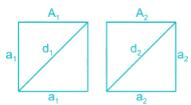Given,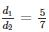Using Pythagoras theorm in any one triangle
⇒ d12 = a12 + a12
⇒ d12 = 2a12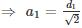Similarly,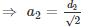∴ The ratio of both squares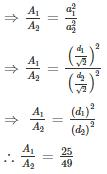QUESTION: 18

The angle of elevation of a ladder leaning against a wall is 30° and the foot of the ladder is 5 m away from the wall. The length of the ladder is

Solution:

Let, the length of the ladder be ‘l’ which is QR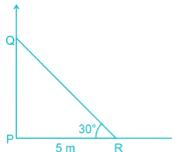Use trigonometric method in given triangle PQR
⇒ cos θ = Base/Hypotenuse
⇒ cos 30° = 5/l
⇒ l = 10/√3 m

QUESTION: 19

The product of two numbers is 1800 and their HCF is 15. The numbers are

Solution:

Let, the two numbers are 15x and 15y
Given,
⇒ (15x) × (15y) = 1800
⇒ xy = 8
Value of co-primes of x and y are (1, 8)
∴ The two numbers are (1 × 15, 8 × 15) = (15, 120)

QUESTION: 20

The difference between simple and compound interests compounded annually on a certain sum of money for 2 years at 2% per annum is Rs. 3. The sum (in Rs.) is:

Solution:

Let, the sum of the money be x. CI is compound interest and SI is Simple interest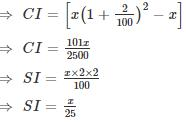Given,
CI – SI = 3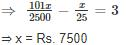QUESTION: 21

In a mixture of 20 litres, the ratio of milk to water is 3 : 2. Another 2 litres of water is added to the mixture. The ratio of milk to water in new mixture is

Solution:

Let, the milk be in given mixture is 3x and water be in given mixture is 2x
According to question,
⇒ 3x + 2x = 20
⇒ x = 4
∴ the milk in given mixture is 12 litres and the water be in given mixture is 8 litres
After adding 2 litres of water in the given mixture, the quantity of water will be (8 + 2) = 10 litres
∴ Ratio of milk to water in new mixture = 12/10 = 6/5 = 6 : 5

QUESTION: 22

Direction: The pie chart given below represents the modes of transport for 1400 officers of the Staff Selection Commission, Kolkata. Study the chart and answer the following questions.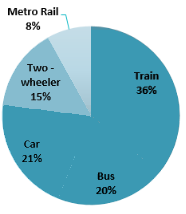Question: The number of officers who go to office by Bus is

Solution:

∵ Total number of officers = 1400
∵ Percentage of officer who go to office by Bus = 20%
∴ Number of officers who go to office by Bus = 20% of 1400 = 280

QUESTION: 23

Direction: The pie chart given below represents the modes of transport for 1400 officers of the Staff Selection Commission, Kolkata. Study the chart and answer the following questions.Question: The number of officers who go to office by Metro Rail is

Solution:

∵ Total number of officers = 1400
∵ Percentage of officer who go to office by Metro Rail = 8%
∴ Number of officers who go to office by Metro Rail = 8% of 1400 = 112
मेट्रो रेल से कार्यालय जाने वाले अधिकारियों की संख्या क्या है?

QUESTION: 24

Direction: The pie chart given below represents the modes of transport for 1400 officers of the Staff Selection Commission, Kolkata. Study the chart and answer the following questions.Question: Write down the difference of officers availing train and the officers availing car as mode of transport?

Solution:

∵ Total number of officers = 1400
∵ Percentage of officer using Cars = 21%
∵ Percentage of officer using trains = 36%
∴ required difference = 36% of 1400 – 21% of 1400 = 15% of 1400 = 210

QUESTION: 25

Direction: The pie chart given below represents the modes of transport for 1400 officers of the Staff Selection Commission, Kolkata. Study the chart and answer the following questions.Question: The ratio of two - wheelers and trains being used as modes of transport is

Solution:

∵ Total number of officers = 1400
∵ Percentage of officer using two - wheelers = 15%
∵ Percentage of officer using trains = 36%
∴ Required ratio = (15% of 1400) : (36% 0f 1400) = 15 : 36 = 5 : 12